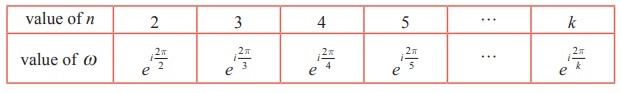Home | | Maths 12th Std | The nth roots of unity

# The nth roots of unity

Mathematics : Complex Numbers: The nth roots of unity

The nth  roots of unity

The solutions of the equation zn = 1 , for positive values of integer , are the roots of the unity.

In polar form the equation zn = 1 can be written as

zn = cos (0 + 2k¤Ç) + sin (0 + 2k¤Ç) = ei2k¤Ç , k = 0, 1, 2,ÔÇŽ..

Using deMoivreÔÇÖs theorem, we find the nth roots of unity from the equation given below:Given a positive integer , a complex number is called an n th root of unity if and only if zn = 1.

If we denote the complex number by ¤ë , thenTherefore ¤ë is an nth root of unity. From equation (1), the complex numbers 1,¤ë,¤ë2 ,... ...,¤ën-1 are nth roots of unity. The complex numbers 1,¤ë,¤ë2 ,... ...,¤ën-1 are the points in the complex plane and are the vertices of a regular polygon of n sides inscribed in a unit circle as shown in Fig 2.45. Note that because the n th roots all have the same modulus 1, they will lie on a circle of radius 1 with centre at the origin. Furthermore, the n roots are equally spaced along the circle, because successive n th roots have arguments that differ by 2¤Ç/n .

The nth roots of unity 1,¤ë,¤ë2 ,... ...,¤ën-1 are in geometric progression with common ratio ¤ë

Therefore 1+ ¤ë + ¤ë2 +ÔÇŽ + ¤ën-1 = 1- ¤ën / 1- ¤ë = 0  since ¤ën = 1 and ¤ë Ôëá 1 .

The sum of all the nth roots of unity is

1+ ¤ë + ¤ë2 +ÔÇŽ + ¤ën-1 = 0

The product of nnth roots of unit is

1¤ë¤ë2 ... ...¤ën-1 = ¤ë0+1+2+3+... ...+(n-1) = ¤ë[(n-1)n]/2

The product of all the nth roots of unity is

1¤ë¤ë2 ... ...¤ën-1 = (-1)n-1Note

(1) All the n roots of nth roots unity are in Geometrical Progression

(2) Sum of the n roots of nth roots unity is always equal to zero.

(3) Product of the n roots of nth roots unity is equal to (-1)n-1 .

(4) All the n roots of nth roots unity lie on the circumference of a circle whose centre is at the origin and radius equal to 1 and these roots divide the circle into n equal parts and form a polygon of n sides.

Example 2.32

Find the cube roots of unity.

Solution

We have to find 11/3 . Let z = 11/3 then z3 = 1.

In polar form, the equation z3 = 1 can be written as

z3 = cos(0 + 2k¤Ç) + i sin(0 + 2k¤Ç) = ei2k¤Ç , k = 0, 1, 2,...Example 2.33

Find the fourth roots of unity.

Solution

We have to find 11/4. Let z =11/4 . Then z4 = 1 .

In polar form, the equation z4 = 1 can be written as

z4 = cos (0 + 2k¤Ç ) + i sin (0 + 2k¤Ç ) = ei2k¤Ç , k = 0, 1, 2,...Note

(i) In this chapter the letter ¤ë is used for nth roots of unity. Therefore the value of ¤ë is depending on n as shown in following table.(ii) The complex number zei╬Ş is a rotation of z by ╬Ş radians in the counter clockwise direction about the origin.

Example 2.34

Solve the equation z3 + 8i = 0 , where z Ôłł C

### Solution

Let z3 + 8i = 0 . Then, we get

z3 = -8i### Example 2.35

Find all cube roots of ÔłÜ3 +

Solution

We have to find (ÔłÜ3 + i)1/3. Let z3 = ÔłÜ3 + i = r (cos╬Ş + i sin╬Ş )### Example 2.36

Suppose z1 , z2, and z3 are the vertices of an equilateral triangle inscribed in the circle |z| = 2. If z1 = 1+ iÔłÜ3 , then find z2 and z3.

### Solution

|z| = 2 represents the circle with centre (0, 0) and radius 2.

Let A, B, and C be the vertices of the given triangle. Since the vertices z1 , z2 , and z3 form an equilateral triangle inscribed in the circle |z| = 2 , the sides of this triangle AB, BC, and CA subtend 2¤Ç/3 radians (120 degree) at the origin (circumcenter of the triangle).

(The complex number z ei╬Ş is a rotation of z by ╬Ş radians in the counter clockwise direction about the origin.)

Therefore, we can obtain z2 and z3 by the rotation of z1 by 2¤Ç/3 and 4 ¤Ç/3 respectively.

Given thatTherefore, z2 = -2, and z3 = 1- iÔłÜ3.

Tags : Definition, Formula, Solved Example Problems , 12th Mathematics : UNIT 2 : Complex Numbers
Study Material, Lecturing Notes, Assignment, Reference, Wiki description explanation, brief detail
12th Mathematics : UNIT 2 : Complex Numbers : The nth roots of unity | Definition, Formula, Solved Example Problems